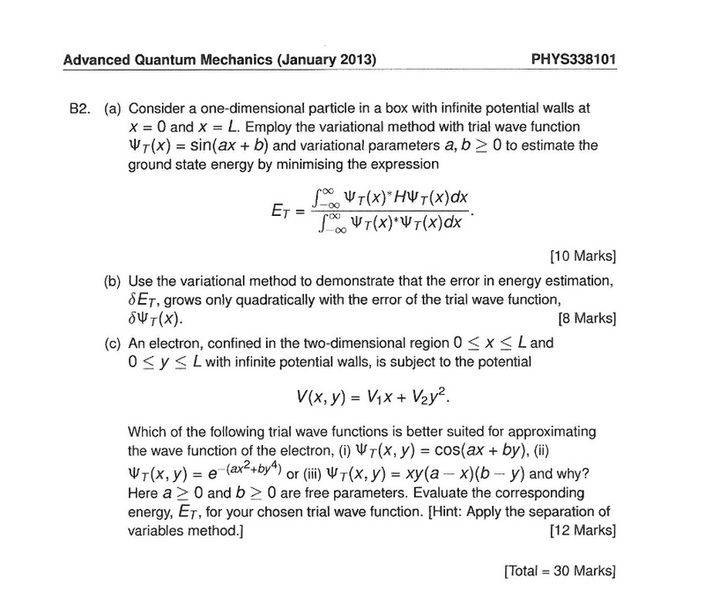# Ground state energy from the variational method

• rwooduk
I know I'll need it!In summary, the problem asks for the wave function for a particle in a box with potential walls at x=0 and x=L. The variational method is used to find the ground state energy, and it is found to be a constant function with energy 0.f

## Homework Statement

Consider a one-dimentional particle in a box with infinite potential walls at x=0 and x=L. Employ the variational method with the trial wave function ΨT(x) = sin(ax+b) and variational parameters a,b>0 to estimate the ground state energy by minimising the expression

$$E_{T}= \frac{\left \langle \Psi _{T}(x) |H |\Psi _{T}(x) \right \rangle}{\left \langle \Psi _{T}(x) |\Psi _{T}(x) \right \rangle}$$

## The Attempt at a Solution

I calculate the denominator first

$$\left \langle \Psi _{T}(x) |\Psi _{T}(x) \right \rangle = \int_{0}^{L} sin^{2}(ax+b) dx$$

I use the trig function

$$sin^{2}A = \frac{1}{2} - cos (2A)$$

then integrate to give

$$= \frac{L}{2} - \frac{1}{2a}sin(2aL+2b)+\frac{1}{2a}sin(2b)$$

then the numerator

$$\left \langle \Psi _{T}(x) |H |\Psi _{T}(x) \right \rangle = \frac{(-i\hbar)^{2}}{2m}\int_{0}^{L}\Psi ^{*}\frac{d^{2}}{dx^{2}} sin(ax+b)dx$$

$$= \frac{\hbar^{2}a^{2}}{2m}\int_{0}^{L}sin^{2}(ax+b)dx$$

which is the same integral as before so gets the same result, therefore we have

$$E_{T}= \frac{\hbar^{2}a^{2}}{2m}\frac{\frac{L}{2} - \frac{1}{2a}sin(2aL+2b)+\frac{1}{2a}sin(2b)}{\frac{L}{2} - \frac{1}{2a}sin(2aL+2b)+\frac{1}{2a}sin(2b)}$$

which gives

$$E_{T}= \frac{\hbar^{2}a^{2}}{2m}$$

this is the bit I'm stuck on, I need to minimise this. if i differentiate with respect to a and set to zero I get a=0.

if there is a potential term it's fine i can do that, but with this question there isnt, any ideas how to minimise it?

as always thanks for any help.

I find it strange that you are expected to use variational method for that problem. The trial function ##\sin(ax+b)## is not constructed to automatically satisfy the boundary condition ##\psi(0)=\psi(L)=0##... Where is this problem taken from?

EDIT: I think the idea of this exercise is to demonstrate that the variational method can not be applied blindly without taking into account the boundary conditions relevant for the problem.

Last edited:
•rwooduk
I find it strange that you are expected to use variational method for that problem. The trial function ##\sin(ax+b)## is not constructed to automatically satisfy the boundary condition ##\psi(0)=\psi(L)=0##... Where is this problem taken from?

EDIT: I think the idea of this exercise is to demonstrate that the variational method can not be applied blindly without taking into account the boundary conditions relevant for the problem.

It's from a past exam paper I'm working through in preparation for exams in January. Here is the complete question:So would you say that a minimisation of the trial wave function is not needed for this problem? How would you make the statement that it can't be minimised or that the boundary conditions play a part? As I mentioned before when there's a potential term involved, such as for the hydrogen atom minimisation is possible as there are two terms in the minimising differentiation, however I can't see how it works in this problem.

Thanks for the reply and any further ideas would be welcome

If I was given this exercise, I would just answer that the problem can't be solved because the trial function is invalid due to boundary conditions. If you apply the variational method here without thinking what you are doing, you get the answer that the minumum energy wavefunction is a constant function ##\psi(x)=C## which has energy 0. However, the constant function does not vanish at ##x=0## and ##x=L##, so it is not an acceptable solution.

•rwooduk
This has had me confused for some time, thanks again for your help!

If you get the boundary conditions right, on the other hand, you fix b and a just gets a set of discrete set of values, where the smallest one gives exactly the ground state wave function - no variations necessary.
That's a weird problem.

If you get the boundary conditions right, on the other hand, you fix b and a just gets a set of discrete set of values, where the smallest one gives exactly the ground state wave function - no variations necessary.
That's a weird problem.

The guy running the course is great and his lectures are awesome but he likes to set "interesting" questions on the exam, it really doesn't help when all you want is questions you have done before and recognise. Part (c) of that question is another example of "why does he do this?", just give me the wave fuction and i can do the problem.. I'm not looking forward to the exam, at all.

Thanks again